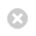Close

The Bartlett Centre for Advanced Spatial Analysis

Home# CASA Working Paper 18021 February 2012

A mathematical challenge: the centre size distribution in a dynamic retail model

The standard retail model can be constructed as follows (cf. Wilson, 1970). Let {Sij} be a matrix of money flows from residents in each zone i to retail centres in each zone j. Let ei be the per capita expenditure in i and Pi the population, so that eiPi is the total retail expenditure leaving i. Let cij be transport costs as before with C as the total transport expenditure. Suppose residents gain a benefit from using centres of a particular size that is proportional to logWj, say, where Wj is the size in floor space of centre j. Let X be the total of such benefits. Then we can maximise an entropy function subject to the constraints that represent our knowledge of the system.

Max S = -ΣSijlogSij (1)

such that

ΣjSij = eiPi (2)

ΣijSijlogWj = X (3)

ΣijSijcij = C (4)

which, after the usual manipulations, leads to

Sij = AieiPiWjαexp(-βcij) (5)

with

Ai = 1/∑kWkαexp(-βcik) (6)

Because the attraction ’end’ is unconstrained, this allows us to calculate

Dj = ΣiSij (7)

which is the total amount of money attracted into a centre. We thus have a model that predicts a key locational variable, Dj.

Authors:  Alan Wilson

Publication Date: 21st February 2012

RPS ID: